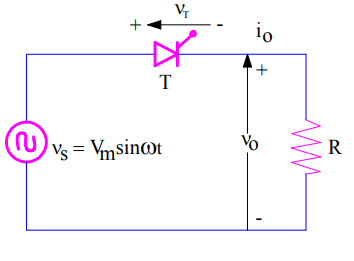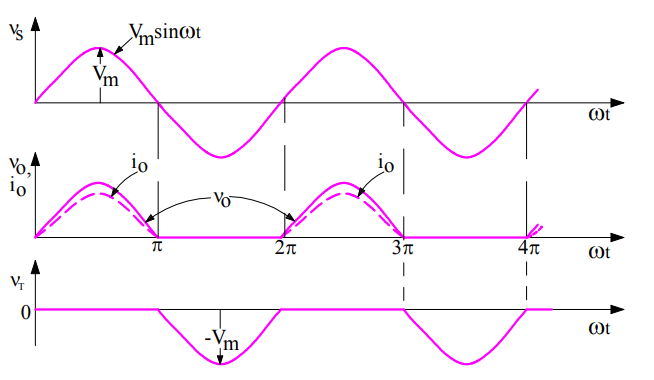# Natural or Line Commutation- Principle with Circuit Diagram

Natural or Line commutation is a Class-F SCR commutation technique in which, a thyristor is turned off due to natural current zero and voltage reversal after every half cycle. This commutation method is only applicable for AC circuit and mostly used in Phase-Controlled Converters, Line-Commutated Inverters, AC voltage controllers and Step-down cycloconverters.

### Principle of Class-F or Natural or Line Commutation:

When an SCR circuit is energized from an AC source, current has to pass through its natural zero at the end of every half cycle. The AC source then applies a negative voltage across cathode and anode terminals of SCR.

As we know that, the required conditions for SCR to turn off are

• Current through SCR must reach below its holding current, and
• SCR must be reversed biased for a time more than its turn off time (tq) so that it may recover the stored charges.

Since, current reaches zero at the end of every half cycle and voltage across the SCR is negative, hence SCR will turn off provided the half time period must be greater than SCR turn off time tq.

### Circuit Diagram of Natural or Line Commutation:

Let us consider a simple circuit as shown in figure below. The circuit is energized by an AC source.Let us also assume that, thyristor T is fired or gated at firing angle equal to zero i.e. ωt = 2nπ where n is 0,1,2,3…..,. Since load is resistive, with zero degree firing angle, the SCR behaves like a diode. During positive half cycle, the SCR will conduct as it is forward biased. The output voltage V0 = (Vs/R) will have same wave shape as that of source Vs. Load current i0 will be in phase with the load voltage v0, hence i0 will have wave shape similar to load voltage v0 & vs.At ωt = π, source voltage vs = 0, load voltage v0 = 0 and load current i0 = 0. Therefore, the current through the SCR becomes zero at this instant and it is reversed biased form ωt = π to 2π. Refer the voltage profile curve VT across thyristor in the above figure. This means, SCR is reversed biased for a time of t = (π/ω). If this time t is more than SCR turn off time tq, the SCR will turn off or commutated. Time t for which SCR is reversed biased is called circuit turn off time tc.

Hence circuit turn off time for Natural or Line or Class-F commutation is tc = (π/w).

### Why it called Natural Commutation?

The commutation of SCR does not involve any external circuit. SCR is commutated just because of the natural reversal of AC load current and source voltage, this is the reason it is called Natural Commutation.

This site uses Akismet to reduce spam. Learn how your comment data is processed.